# CloudTracer Latency Anomaly Events

The cloudtracer latency anomaly event monitors the latency metric between devices and configured hosts. The events are designed to alert the user when the latency between a device and a configured host is outside of recent historical bounds.

Figure 1 is a sample event view for one of these events between the device with hostname `Oslo` and the cloudtracer host endpoint `www.bbc.co.uk`.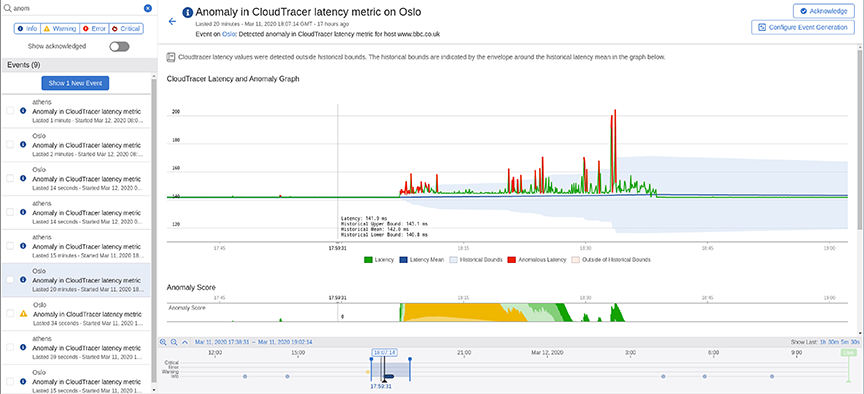Figure 1. Anomaly Event View

Figure 2 explains various stages of this event.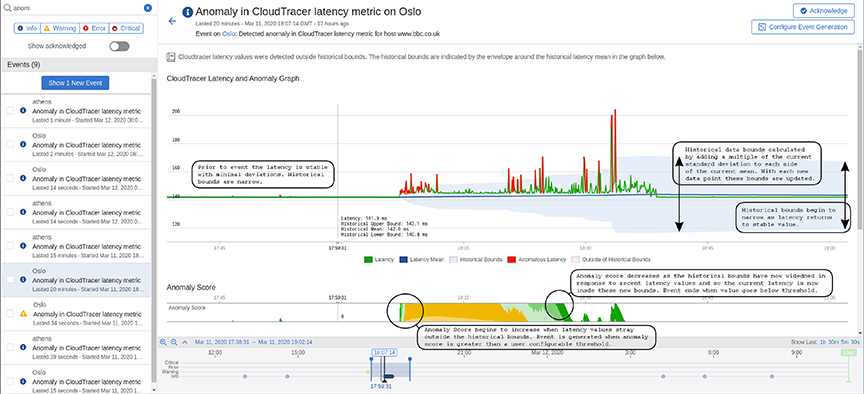Figure 2. Anomaly Event View Overlay

Prior to this event in Figure 2, the latency metric (green line in upper graph) is stable with minimal deviations. The historical bounds (blue shaded region) that determine when the metric is in a normal state has a small range with both the upper and lower bounds near the historical mean (dark blue line). The historical bounds are computed by adding and subtracting a fixed multiple of the current latency standard deviation to the current mean.

The anomaly score starts to increase from zero when the latency value strays outside of the historical bounds. The latency values that are outside the bounds are highlighted in red. The anomaly score is the total number of standard deviations outside the historical bounds. The anomaly score is the positive cumulative sum of the number of standard deviations outside of the historical bounds. For example, if the bounds are set as 3 standard deviations outside of the mean and we get a value of the latency that is 5 times the standard deviation away from the mean, the anomaly score will increase by 2. If the next latency value was 1.5 times the standard deviation outside of then mean then we would subtract 1.5 from the anomaly score. The anomaly score therefore keeps track of the cumulative deviation of the latency outside of the historical bounds. It is bounded below by zero.

Figure 3 provides a detailed explanation on computing the anomaly score.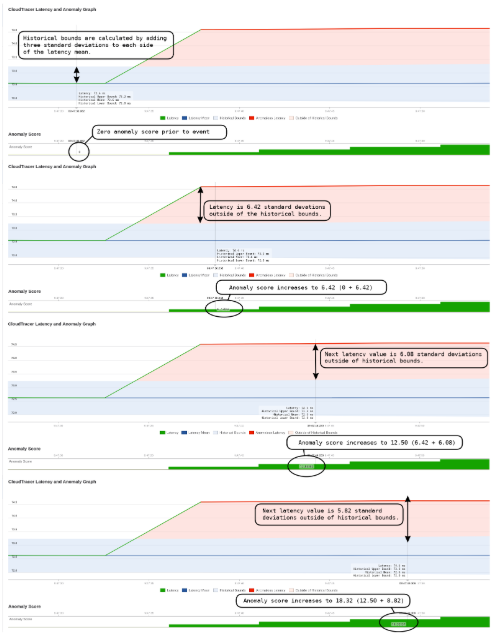Figure 3. Anomaly Score Computation

The event is generated when the anomaly score exceeds a threshold for a set period of time.

Note: You can configure the threshold and time duration in the event configuration rules.

The anomaly score starts to decrease when the latency values are inside the historical bounds. The historical bounds have increased based on recent deviations in latency which makes the system less sensitive than prior to the event. The event ends when the anomaly score is below the threshold for a set period of time.

Figure 4 provides a detailed explanation of the anomaly score decreasing when an event ends.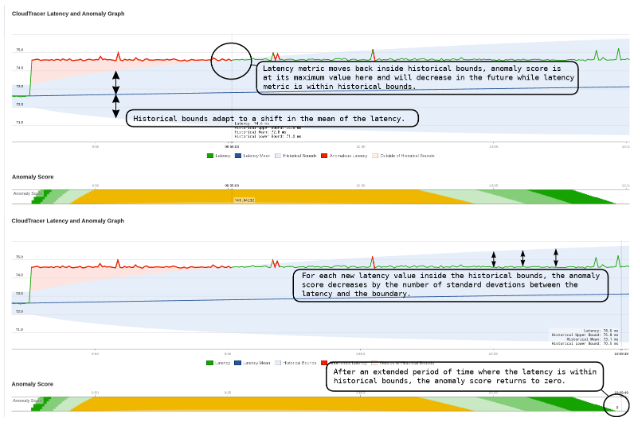Figure 4. Decreasing of Anomaly Score

At the end of the time range, historical bounds are narrowing as the latency has now returned to a stable value with minimum deviations. The history needs approximately six hours to have negligible impact on the statistics and bounds.

This screen also provides the following additional metrics of this event (see Figure 5):

• The other CloudTracer metrics are displayed for this device and host pair

• The latency metric between other devices and this host

• The latency metric between this device and other hosts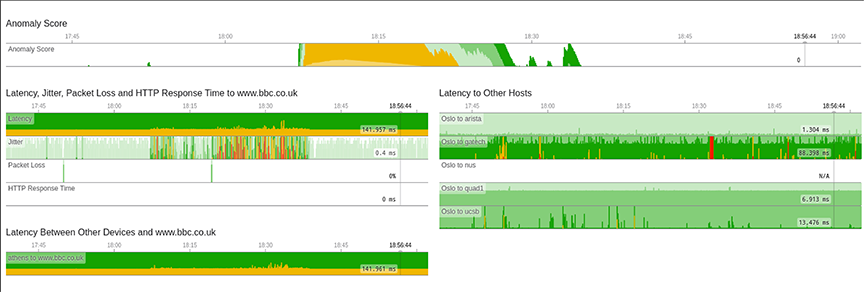Figure 5. CloudTracer Event Additional View